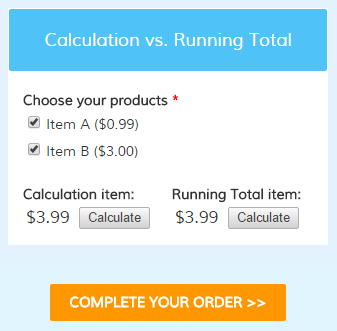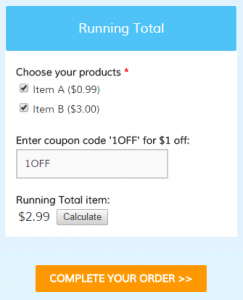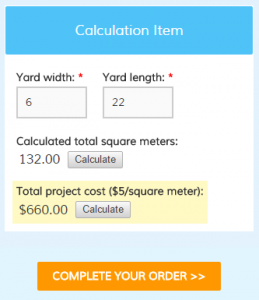# Calculation Item or Running Total Item: Differences & Use

Making forms that calculate a value, like order forms and registration forms, will use the Calculation item or Running Total item to show the calculated total. These items appear to be identical on the form but they are very different in actual use. Each has its best use and while it may be possible to use one for the other, many problems can be avoided with a little up-front knowledge.

## The BasicsThe Running Total item is used to display the actual order total when creating order forms. Regardless of how complex your form may be, the Running Total item automatically keeps track of all selected quantities, percentage calculations, coupon codes, and any other factors that affect the order total.

The Calculation item is used to process a custom equation in a single step and may use an item value, number, or score as the equation values. The value that results is the result of the equation and can be formatted as currency but does not have to be.

##When to use a Running Total item:

If your form is an order form and contains pricing items, use the Running Total item. This item is a way to display the actual current value on the form before the visitor reaches the Order Summary page.

The Running Total item automatically calculates all of the order form items so you don’t have to manually enter the equation. If you find yourself using a Calculation item that only adds up all of your order items, consider replacing it with the Running Total item.

## When to use a Calculation item:

If your form uses numbers for sizes, distances, temperature, scores, or anything other than money, use a Calculation item. If your equation contains anything other than the basic sum of the values, the Calculation item will let you specify that custom equation.

For example, if you need to calculate the number of square meters in your lawn you can make a form containing fields for the dimensions, then add your Calculation item to multiple length x width.

##How to calculate money:

Sometimes you have a need to perform a calculation on your pricing item. Continuing with the example above, to calculate the total cost for servicing the lawn you would create your Calculation item to calculate the area then multiply the area x cost per meter to provide the total cost.

You can then add the calculated cost to the order total by editing the Calculation item and enabling the setting Include in Order Total.